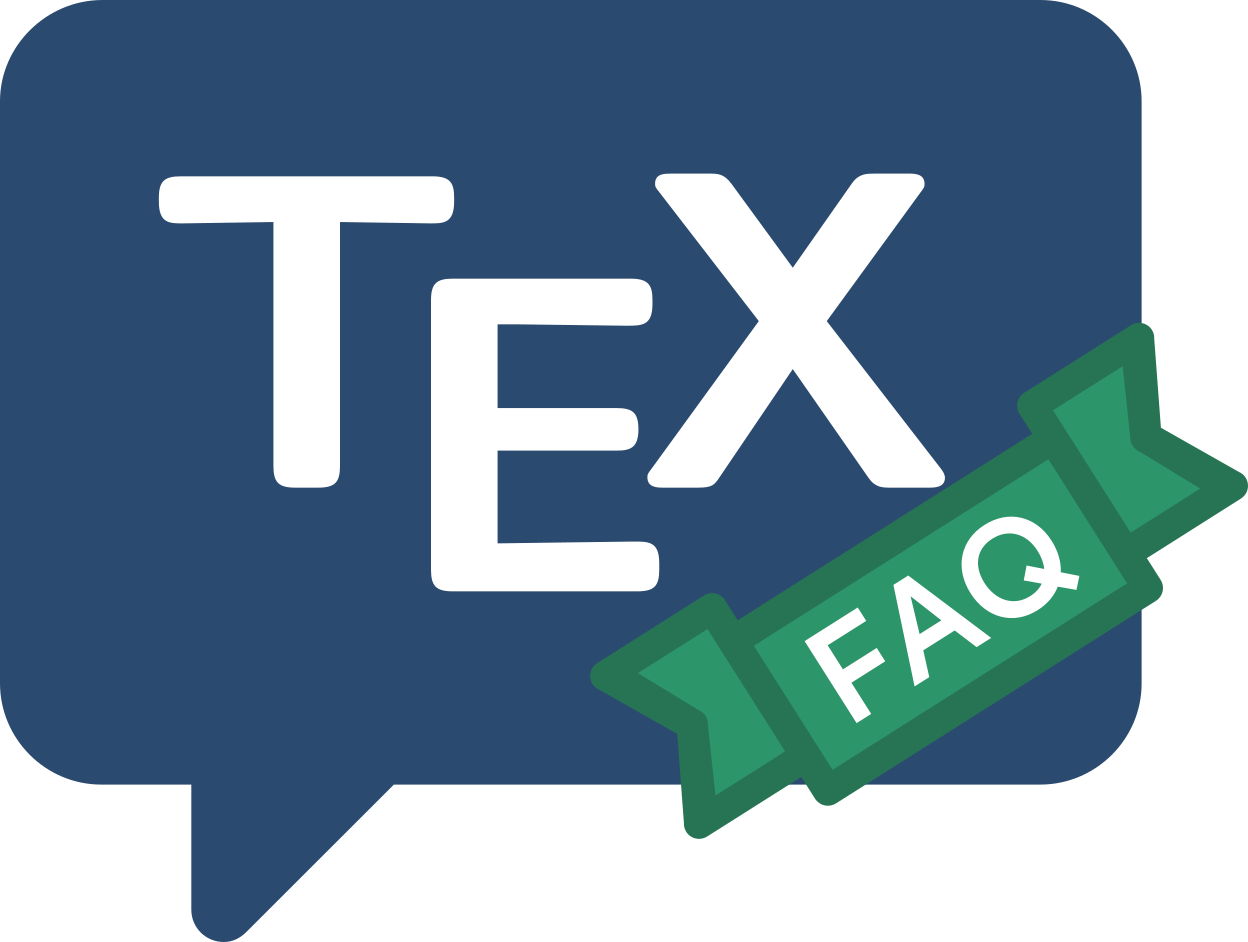Frequently Asked Question List for TeX

# Proof environment

It was long thought impossible to make a proof environment which automatically includes an “end-of-proof” symbol. Some proofs end in displayed maths; others do not. If the input file contains ...\] \end{proof} then LaTeX finishes off the displayed maths and gets ready for a new line before it reads any instructions connected with ending the proof, so the code is very tricky. You can insert the symbol by hand, but the (apparently) original “automatic” solution came with Paul Taylor’s QED.

Nowadays, the ntheorem package now solves the problem for LaTeX users: it provides an automatic way of signalling the end of a proof.

The AMSLaTeX package amsthm also provides a proof environment that does the job; though you need to insert a \qedhere command if the proof ends with a displayed equation:

\begin{proof}
text...
\begin{equation*}
maths... \tag*{\qedhere}
\end{equation*}
\end{proof}


The \tag*{\qedhere} construction may be used in any of AMSLaTeX’s numbering environments.

FAQ ID: Q-proof
Tags: math